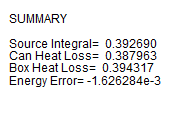﻿ User Guide > Using FlexPDE in Three-Dimensional Problems > Integrals in Three Dimensions

# Integrals in Three DimensionsIn three-dimensional problems, volume integrals may be computed over volume compartments selected by region and layer.

 • Result = VOL_INTEGRAL()

Computes the integral of the integrand over the entire domain.

 • Result = VOL_INTEGRAL(, )

Computes the integral of the integrand over all layers of the specified region.

 • Result = VOL_INTEGRAL(, )

Computes the integral of the integrand over all regions of the specified layer.

 • Result = VOL_INTEGRAL(, , )

Computes the integral of the integrand over the compartment specified by the region and layer names.

 • Result = VOL_INTEGRAL(, , )

Computes the integral of the integrand over the compartment specified by the region and layer numbers.

Surface integrals may be computed over selected surfaces.  From the classification of various qualifying names, FlexPDE tries to infer what surfaces are implied in a surface integral statement. In the case of non-planar surfaces, integrals are weighted by the actual surface area.

 • Result = SURF_INTEGRAL()

Computes the integral of the integrand over the outer bounding surface of the total domain.

 • Result = SURF_INTEGRAL(, {, } )

Computes the integral of the integrand over all regions of the named extrusion surface. If  the optional <layer_name> appears, it will dictate the layer in which the computation is performed.

 • Result = SURF_INTEGRAL(, , {, } )

Computes the integral of the integrand over the named extrusion surface, restricted to the named region.  If  the optional <layer_name> appears, it will dictate the layer in which the computation is performed.

 • Result = SURF_INTEGRAL(, , )

Computes the integral of the integrand over all surfaces of the compartment specified by the region and layer names.  Evaluation will be made inside the named compartment.

 • Result = SURF_INTEGRAL(, {, } )

Computes the integral of the integrand over all layers of the sidewall generated by the extrusion of the named base-plane curve. If the optional <region name> argument appears, it controls on which side of the surface the integral is evaluated.  Portions of the surface that do not adjoin the named layer will not be computed.

 • Result = SURF_INTEGRAL(, , {, } )

Computes the integral of the integrand over the sidewall generated by the extrusion of the named base-plane curve, restricted to the named layer.  If the optional <region name> argument appears, it controls on which side of the surface the integral is evaluated. Portions of the surface that do not adjoin the named layer will not be computed.

Note: The example problem "Samples | Usage | 3D_Integrals.pde" demonstrates several forms of integral in a three-dimensional problem.

Let us modify our Canister problem to contain a heat source, and compare the volume integral of the source with the surface integral of the flux, as checks on the accuracy of the solution:

TITLE 'Heat flow from an Insulating Canister'

COORDINATES

Cartesian3

VARIABLES

Phi                { the temperature }

DEFINITIONS

K = 1                { default conductivity }

R = 0.5        { blob radius }

S = 0

EQUATIONS

EXTRUSION

SURFACE 'Bottom'  z=-1/2

LAYER 'underneath'

SURFACE 'Can Bottom'  z=-1/4

LAYER 'Can'

SURFACE 'Can Top'  z=1/4

LAYER 'above'

SURFACE 'Top'        z=1/2

BOUNDARIES

REGION 1 'box'

START(-1,-1)

VALUE(Phi)=0        LINE TO (1,-1)

NATURAL(Phi)=0        LINE TO (1,1)

VALUE(Phi)=1        LINE TO (-1,1)

NATURAL(Phi)=0        LINE TO CLOSE

REGION 2        'blob'        { option: could be LIMITED }

LAYER 2 k = 0.001        { the canister only }

S = 1                        { still the canister }

START 'ring' (R,0)

ARC(CENTER=0,0) ANGLE=360 TO CLOSE

PLOTS

GRID(y,z) on x=0

CONTOUR(Phi) on x=0

ELEVATION(Phi) FROM (0,-1,0) to (0,1,0)

SUMMARY

REPORT(Vol_Integral(S,'blob','can')) AS 'Source Integral'

AS 'Can Heat Loss'

AS 'Box Heat Loss'

AS 'Energy Error'

END

The contour plot is as follows:The summary page shows the integral reports:Note: The "Integral" reported at the bottom of the contour plot is the default Area_Integral(Phi) reported by the plot processor.